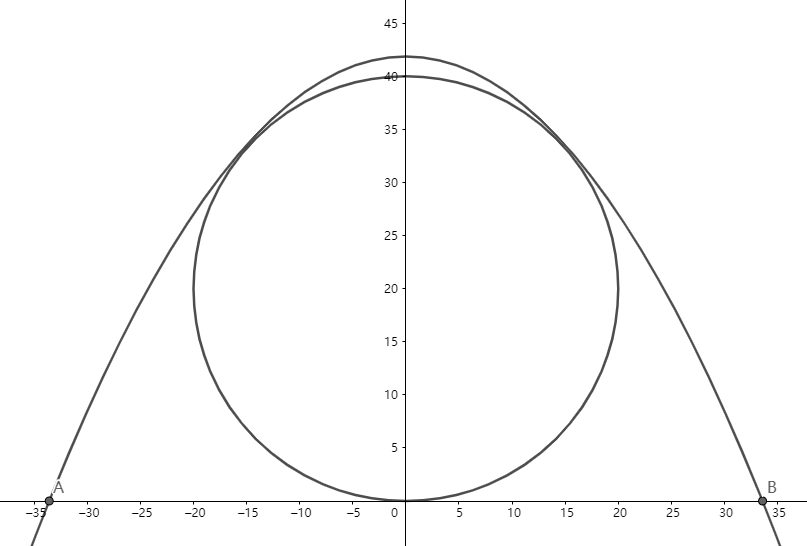# Reach for the Summit - P-S1-A4A pipe with diameter $D=0.2 \text{ m}$ is put on the horizontal ground, and Ant-Man wants to jump over it to practice his strength. If Ant-Man is initially on the ground, and he can start to jump anywhere, what is the minimum initial velocity he should have to jump over the pipe? Let $v_m \text{ m/s}$ be the minimum velocity. Submit $\lfloor 1000v_m \rfloor$.

Assumptions:

• Ignore air resistance.
• He can be treated as a mass point.
• Take gravitational acceleration $g=10 \text{ m/s}^2$.

Reach for the Summit problem set - Physics

×